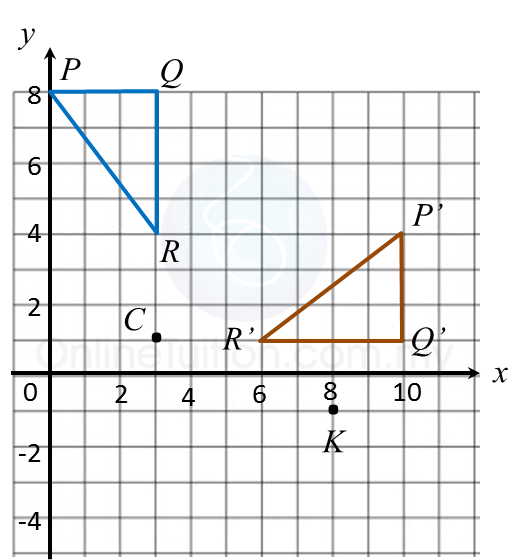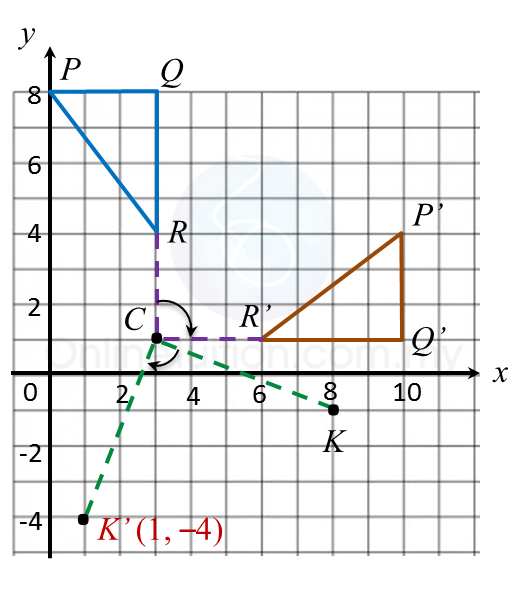# 11.2.3 Isometric Transformations, PT3 Focus Practice

    
        
         
Question 7:
         
Diagram in the answer space, shows two polygons, M and M’, drawn on a grid of equal squares with sides of 1 unit. M’ is the image of M under a reflection.
         
(a) Draw the axis of reflection.
         
(b) Mark the image of P under the same reflection.
         
(c) Draw the image of M under reflection in the x-axis.
         

     Answer
:Solution:Question 8:
         
On diagram in the answer space, triangle P’Q’R’ is the image of triangle PQR under a rotation about centre C.
         
(a) State the angle and direction of the rotation.
         
(b) K’is the image of point K under the same rotation.
         
Mark and state the coordinates of K’.
         

     Answer
:Solution:(a) ∆ P’Q’R’ is the image of ∆ PQR under a clockwise rotation of 90o.
            
(b) Image of K = (1, –4).